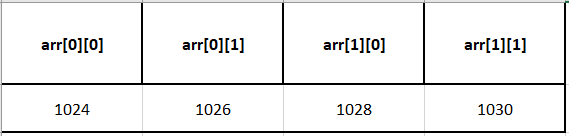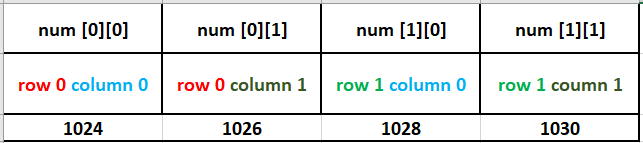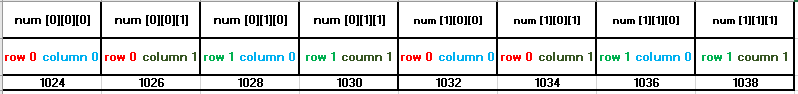In this tutorial, we are going to learn about Multi Dimensional Arrays in C.

## C Multi Dimensional Arrays:

C provides the ability to create multi dimensional arrays. Below are a few examples of multi dimensional arrays:

Syntax:

``````data_type array_name[size_1][size_2][size_3].......[size_n];
//where size_1 to size_n from the dimensions of the declared array``````

Examples:

``````int arr_2d; //an example for a two-dimensional array
int arr_3d; //an example for a three-dimensional array
int arr_4d; //an example for a four-dimensional array``````

Two-dimensional and three-dimensional arrays are the most common types of multi-dimensional arrays.

A two-dimensional array is used to represent the values in the form of rows and columns.

## Two Dimensional Array:

In a two-dimensional array, two sizes are provided. The first subscript represents the row size while the second subscript represents the column size. The format of declaring a two-dimensional array is Below is an example of a two-dimensional array:

``int arr;``

In the above declaration, arr is the name of the array, which contains 2 rows with 2 columns each that represent a block of 4 consecutive storage locations.
Where,

arr and arr represent the elements in the 0th row
arr and arr represent the elements in the 1st rowEach element of a two-dimensional array is accessed by its row and column index.
Let us consider an example of a two-dimensional array:

```int arr; //declares an array with 2 rows and 2 columns
representing a block of 4 consecutive storage locations```In the above example, the array variable arr contains the base address of the entire array. Let us assume the base address as some random number: 1024.

The formula for finding the location of the element in the ith row and jth column is :

``````Address of the ith row and jth column element
= *(base_address + i) + j
= base_address + i * total_columns * scale_factor + j * scale_factor``````

Using the above formula, the location of arr can be calculated as below:

``````*(arr + 0) + 1 //where 0 is the row's index and 1 is the
column's index = 1024 + 0 * total_columns * [scale_factor] + 1 * [scale_factor]
= 1024 + 0 * 3 * 2 + 1 * 2
= 1024 + 0 + 2
= 1026``````

In the above line, 2 is the size of the data type int in 16-bit machines. 2 is also referred to as a scale factor.

The scale factor is automatically calculated by the system, which is a value representing the size of the data type of the array.

Note: In the below program, the code snippet *(num + i) + j is used to find the address of ith row and jth column, and the code snippet * (*(num + i) + j) is used to find the value at ith row and jth column

## Three Dimensional Array:

three dimensional array can be visualised as an array of two-dimensional arrays. The 3-D array can also be visualized as pages containing a 2-D array with elements in rows and columns.

Below is an example of a three-dimensional array:

Syntax:

``data_type array_name[size_1][size_2][size_3];``

Example declaration:

``int arr;``

For example, the above 3-D array can be visualized as 2 pages, each containing a 2-D array of 2 rows and columns each, totaling 8 elements, which are stored in consecutive storage locations.## Initialization of arrays:

The elements of an array can also be initialized with the values during declaration instead of reading them by the I/O functions.

Note that an array can be initialized in its declaration only. The lists of values should be enclosed within braces. The values should be separated by commas and must be constants or constant expressions.

A one-dimensional array can be initialized as,

int a = {10, 20, 30, 40, 50};

The values within the braces are scanned from left to right and assigned to aaa, a, and a respectively.

two-dimensional array can be initialized as,

int a = {{10, 20}, {30, 40}};

Here, the values within the inner braces are assigned as elements to each row. If the number of values initialized for an array is less than the size mentioned, the missing elements are assigned to zero.

For example in

int a = {{1, 2}, {3, 4, 5}};

the elements 1 and 2 are stored in aa of the 0th row and elements 3, 4 and 5 are stored in aa and a of the 1st row respectively. All the other elements are initialized to zero.

In the example, int a = {1, 2, 3, 4, 5};, the values or elements 1, 2, 3, 4 and 5 are assigned starting from left-side to aaaa and a respectively.

## Some Special Points:

Below are some important points to remember regarding arrays:

• An array is a derived data type.
• It can store one or more elements of the same data type.
• An element in an array can be referred to as arr[index], where arr is the name of the array and an index is an integer constant that specifies the location of the element in the array.
• An array subscript (index) always starts at zero. Hence, it is said that the array uses zero-based addressing. The last element’s index is always size-1.
• Elements within an array are stored in consecutive memory locations.
• The array name holds/stores the starting memory location of the array, this address is known as the base address of the array.
• Arrays can be one-dimensional or multi-dimensional.
• Array declaration must contain the size without fail.
• The array size must be of integer type which is greater than zero.
• In a multi-dimensional array, each dimension uses a separate pair of square brackets.
• It is a good programming practice to mention the size using symbolic constants which facilitate easier program maintenance.
• It is illegal to refer to the elements that are out of the array bounds. For an array, a, the array bounds are from a to a only. The subscript or index values other than values between 0 to 9 are illegal hence will throw an error saying the array access is out of bounds.
• In C the compiler does not check on the bounds of an array, hence we will see an error only during execution.

## REFERENCES:

Happy Learning 🙂

### About the Author: Hemanth Krishna Vineel RamayanamI’m an Undergraduate Student at the Indian Institute of Information Technology, Design, and Manufacturing, Kurnool, Andhra Pradesh. I belong to the CSE domain.